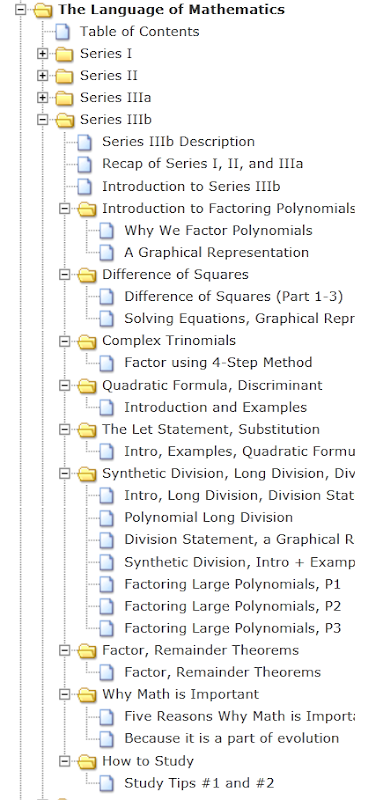| | | | |

## Thursday, February 23, 2012

### Series IIIb Description: What's Contained in this Series

This series is a continuation of Series IIIa.

Series IIIa and IIIb give you the power to be able to factor and solve polynomials of any degree. Factoring techniques included in this series are: the Difference of Squares, Complex Trinomial Factoring (4-step method), Quadratic Formula including The Discriminant, and Synthetic Division.

In addition to the above, we also do an in-depth discussion of The Division Statement, Polynomial Long Division, look at the graphical meaning of factoring polynomials, use Let Statements and Substitution to factor polynomial and non-polynomial functions, and discuss The Remainder and The Factor Theorems.

Extras included in this series are a look at Why Math is Important and two tips on how to improve your study habits.

Full list and links for all the videos contained in the series are provided below and are available at: Table of Contents: The Language of Mathematics - Series IIIb.

See Videos Available for Download for information on the torrent for this series.

For ease of reference, included below is the Table of Contents and the expanded image of the tree menu provided in the left column of this site. Specific topics can be found through the Index (left column).

Section 1: Introduction to Factoring Polynomials
Section 2: Difference of Squares
Section 3: Complex Trinomials: Factoring using the 4-Step Method
Section 4: The Quadratic Formula and The Discriminant
Section 5: The Let Statement, and Substitution (with Quadratic Formula)
Section 6: Synthetic Division, Polynomial Long Division, and The Division Statement
Section 7: The Remainder Theorem and The Factor Theorem
Section 8: Why Math is Important
Section 9: Study TipsFrom Series IIIb Tree Menu - The Language of Mathematics